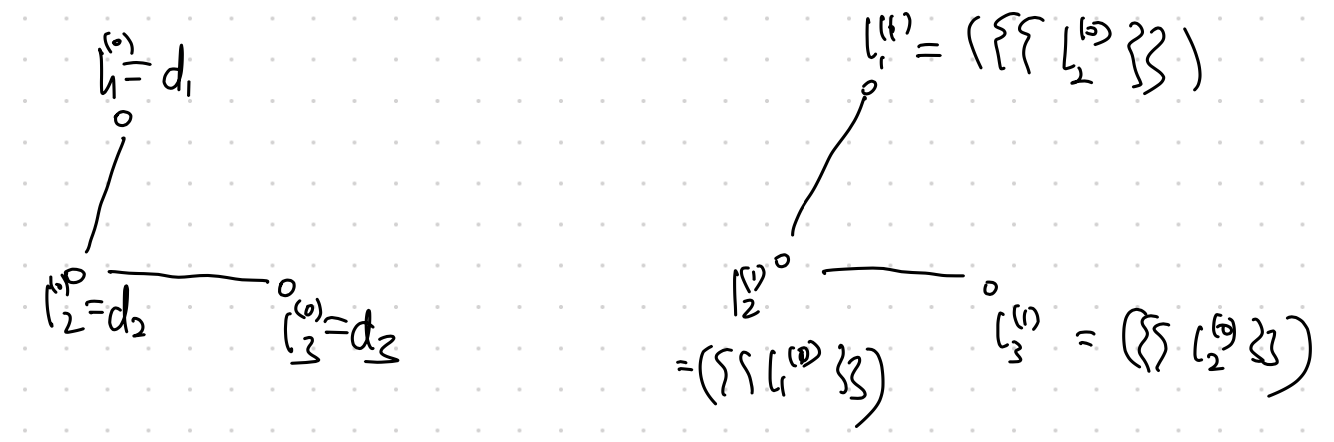The Weisfeiler-Lehman kernel is an iterative integration of neighborhood information.

We initialize the labels for each node using its own node degree. At each step, we take the neighboring node degrees to form a . At step $K$, we have the multisets for each node. Those multisets at each node can be processed to form an representation of the graph which is in turn used to calculate statistics of the graph.Iterate $k$ steps

This iteration can be used to test if two graphs are isomorphism1.

Planted: by ;